## 6457 Reputation

15 years, 188 days
University of British Columbia
Associate Professor Emeritus

## Mix and match...

As I understand it, the system in your worksheet has 9 parameters and 3 values for each.
Allowing all possible choices of a value for each parameter will produce 3^9 = 19683 possibilities, which maybe is a bit much.  But you could try something like this (I'll do it with 4 parameters):

`> As:= map2(`=`,a, [1,2,3]);   Bs:= map2(`=`,b, [4,7, 9]);   Cs:= map2(`=`,c, [3, 1, 4]);   Ds:= map2(`=`,d, [4,6,7]);   T:= combinat[cartprod]([As,Bs,Cs,Ds]);   N:= mul(nops(s),s=[As,Bs,Cs,Ds]);   cases:= [seq](T[nextvalue](), dummy=1..N);`

## Quiver?...

The word Quiver can mean several different things (see http://en.wikipedia.org/wiki/Quiver_%28disambiguation%29.  If you mean plotting a two-dimensional vector field with arrows indicating direction and magnitude, try fieldplot in the plots package.

## Eigenvectors...

In general, there is no reason that eigenvectors should have any particular normalization: any nonzero scalar multiple of an eigenvector is an eigenvector.  Especially for matrices containing symbolic parameters, normalizing the eigenvector may cause more complicated outputs.  For matrices of floats, Maple does normalize the eigenvectors.  However, note that different eigenvectors are not usually orthogonal unless you have a real symmetric matrix (or more generally a normal matrix).

If you do want normalized eigenvectors, you can try something like this:

> with(LinearAlgebra):
A:= <<1,2>|<3,4>>;
E, V:= Eigenvectors(A, output=list);
V:= Matrix(Normalize~([Column(V,1..-1)]));

## Autoexec...

It looks to me like groups for which autoexecute is set have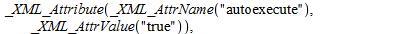So it ought to be possible to write a procedure that would read a worksheet file into an XML tree (using Worksheet:-ReadFile), look for a group with a certain marker, add or remove the attribute, and write the modified tree back to the worksheet file (using Worksheet:-WriteFile).  Here's my rather crude attempt at it:

```SetAutoExecute:= proc(Filename::string, marker::string, tf:: boolean := true)local i,R,C,newC,Rp,tfs;uses XMLTools, Worksheet;R:= ReadFile(Filename);tfs:= convert(tf,string);try  for i from 1 do    C:= GetChild(R,i);    if has(C,marker) then       if HasAttribute(C,"autoexecute") then newC:= SubsAttribute(C,"autoexecute",tfs)      else newC:= AddAttribute(C,"autoexecute",tfs)      end if;      Rp:= ReplaceChild(i=newC , R);      WriteFile(Filename,Rp);      break    end if;                  end do;catch "improper op or subscript selector": error "Did not find %1", marker;end try;end proc;
```

The first argument (Filename) is the name of a worksheet file.  The second is the marker, which should be a string that appears as a text region in the group you are interested in.  The third is true or false (this is optional: default is true).  The procedure will search for the first occurrence of the marker, mark the group it is in as autoexecute or (if the third argument is false) non-autoexecute.

## generating function...

Do you mean you want to derive the generating function for the Laguerre polynomials from the differential equation?  By the way, that web page is wrong: it should be an ordinary generating function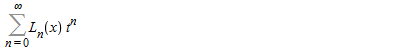not an exponential generating function with n! in the denominator.

I don't know if that is possible.  I can derive a partial differential equation for the generating function, but Maple doesn't seem able to find the desired solution.

The Laguerre polynomial Ln(x) is the solution of the differential equation x y" + (1-x) y' + n y = 0 with initial conditions y(0) = 1 (the differential equation has a regular singular point at x=0 and there is only a one-parameter family of solutions that have finite limits at 0).

Let the generating function be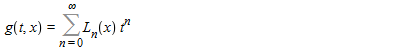The initial conditions say

> g(t,0) = sum(t^n*1, n=0..infinity);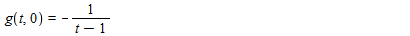Sum the first and second terms from n=0 to infinity, and you get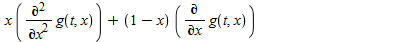But for the third term, noting that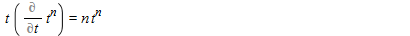you get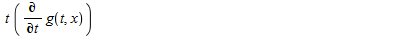So g should satisfy the PDE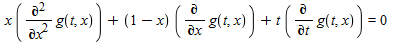pdsolve can solve the PDE, but not with the boundary condition g(t,0) = 1/(1-t).

Here's a different approach.  Maple has the LaguerreL function.  It knows that this is a solution of the Laguerre differential equation:

> dsolve(x*diff(y(x),x\$2) + (1-x)*diff(y(x),x) + n*y(x));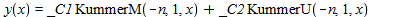> convert(%, LaguerreL);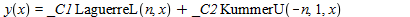And it knows a power series expansion for this:

> convert(LaguerreL(n,x), FormalPowerSeries,x);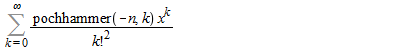Actually the sum should be for k from 0 to n because pochhammer(-n,k) = 0 if n is an integer and k > n: after all, LaguerreL(n,x) is supposed to be a polynomial of degree n in x.  So the generating function should be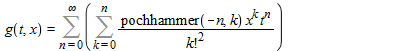Interchange the order of summations and you have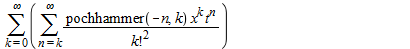Maple needs a little help to do the sum.  We need to use an identity relating pochhammer(-n,k) to pochhammer(k+1,n-k).

> convert(pochhammer(-n,k)/pochhammer(k+1,n-k), factorial) assuming n::posint, k::posint, n>=k;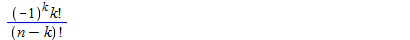So now our double sum becomes

> sum(sum( pochhammer(k+1,n-k)*(-1)^k/(k!*(n-k)!) * x^k * t^n, n=k..infinity), k=0..infinity);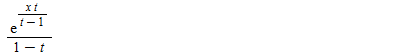## Solutions may be lost...

If your solution is not one of those returned, it looks like the "solutions may be lost" warning was accurate: solutions really were lost.

We might be able to give better advice if you showed us the actual set of equations.

## Expression versus function...

Probably a more serious error: in the definition of qd2 you're using qd3 as a function, i.e. you have qd3(t), but the definition of qd3 makes it an expression, not a function.

## Nonlinear systems...

The standard way to solve systems of equations numerically is using fsolve.  For example:

> fsolve({x+exp(-x)+y^3=0, x^2+2*x*y-y^2+tan(x)=0});

{x = -.7111621793, y = -1.098398307}

However, if you really want to see Newton's method in action, you can do something like this.

> F:= X -> <X+exp(-X)+X^3, X^2+2*X*X-X^2+tan(X)>;
J:= unapply(VectorCalculus:-Jacobian(F(X),[X,X]),X);
Newt:= X -> evalf(X - J(X)^(-1) . F(X));
P:= <-1,0>;
for i from 1 to 10 do P[i]:= Newt(P[i-1]) end do;

For more, you might look at this Maple worksheet.

## details?...

I think we'd need to know more of the details of your problem to be able to suggest a solution.  What precisely do you mean by "i have a function in seri's"?

## three independent variables...

Sorry, the numeric solver of pdsolve allows only two independent variables.

## sum of expressions...

Normally you don't add two plots, you plot the sum of two expressions.
In your case, you have one expression xlam(theta)*A for theta = 0 .. 2*Pi and another,
xlam(theta)*(Sum(B((Rm+Rs)*(1/2), theta), n = 1 .. 100)), for theta = 0 .. 2*Pi/p.  You didn't say what p is, but I presume it's a positive constant.  So you can try

plot(xlam(theta)*A + xlam(theta)*(Sum(B((Rm+Rs)*(1/2), theta), n = 1 .. 100)), theta = 0 .. min(2*Pi, 2*Pi/p));

## define...

This seems to work.

`> interface(imaginaryunit=i): unitquats:= {I,J,K}: unitquat := identical~(unitquats): define(M,'multilinear','flat','identity'=1, M(a::nonunit(freeof(unitquats))*b::unitquat,c::algebraic)=a*M(b,c), M(a::algebraic,c::nonunit(freeof(unitquats))*d::unitquat)=c*M(a,d), M(a::freeof(unitquats),b::algebraic)=a*b,M(a::algebraic,b::freeof(unitquats))=a*b, M(I,I)=-1, M(I,J)=K,  M(I,K)=-J, M(J,I)=-K, M(J,J)=-1, M(J,K)=I, M(K,I)=J,  M(K,J)=-I, M(K,K)=-1);`

For example:
> M(a+b*x*I + c*x^2*J, d*I + x*K);Note, however, that for several factors you must multiply two at a time, e.g. M(M(I,J),K) rather than M(I,J,K).

## dcoeffs...

I'm not sure I understand.  After substituting b=0, there is no term indiff(y(x), x, x).  Do you want to return coefficients of terms that do not exist?  If so, there are infinitely many ...

If you know what terms you want coefficients of, you could try using coeff. A complication is that e.g. diff(y(x),x,x) actually contains diff(y(x),x), which causes trouble if you're looking for coefficients of diff(y(x),x).  But you can work around that by converting from diff to D notation.  For example:

` > Y := subs(b=0, X):   YD := convert(Y,D):   seq(coeff(YD,(D@@2)(y)(x),j),j=2..1,-1),coeff(YD,D(y)(x));`

a, 0, c

## finding text in 2D Math input...

I assume you mean you have a worksheet or document containing 2D Math input which you want to search.  Unfortunately, the help page ?worksheet,documenting,commonactions states

The Find feature does not search content in 2-D math notation.

My suggestion is this:

1) save a temporary copy under a different name (just to be safe)
2) in that copy,  choose Select All, then Format, Convert To, 1D Math Input.  This will change all the 2D Math Input (as well as text) to 1D Math Input.
3) Choose Edit, Find/Replace, and find your text.
4) Edit, Undo Convert To

If it's 2D Math output that you want to search, that might be more challenging, because Convert To doesn't seem to affect it.  One thing you can do is copy an individual output region and paste it into a 1D Math input region, where it becomes 1D Math input.  But I don't know what you can do if you want to search all the outputs in a whole worksheet.

## Rank...

I'm not familiar with contrallability matrices, but if you have a Matrix, its rank can be computed with LinearAlgebra:-Rank.

﻿